Courses

# Test: Digital Transmission

## 20 Questions MCQ Test RRB JE for Electronics & Communication Engineering | Test: Digital Transmission

Description
This mock test of Test: Digital Transmission for Electronics and Communication Engineering (ECE) helps you for every Electronics and Communication Engineering (ECE) entrance exam. This contains 20 Multiple Choice Questions for Electronics and Communication Engineering (ECE) Test: Digital Transmission (mcq) to study with solutions a complete question bank. The solved questions answers in this Test: Digital Transmission quiz give you a good mix of easy questions and tough questions. Electronics and Communication Engineering (ECE) students definitely take this Test: Digital Transmission exercise for a better result in the exam. You can find other Test: Digital Transmission extra questions, long questions & short questions for Electronics and Communication Engineering (ECE) on EduRev as well by searching above.
QUESTION: 1

### A linear delta modulator is designed to operate on speech signals limited to 3.4 kHz. The sampling rate is 10 time the Nyquist rate of the speech signal. The step size δ  is 100 mV. The modulator is tested with a this test signal required to avoid slope overload is

Solution: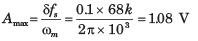QUESTION: 2

### Consider a linear DM system designed to accommodate analog message signals limited to bandwidth of 3.5 kHz. A sinusoidal test signals of amplitude Amax = 1 V and frequency fm = 800 Hz is applied to system. The sampling rate of the system is 64 kHz. Que: The minimum value of the step size to avoid overload is

Solution: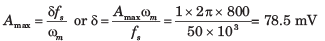QUESTION: 3

### Consider a linear DM system designed to accommodate analog message signals limited to bandwidth of 3.5 kHz. A sinusoidal test signals of amplitude Amax = 1 V and frequency fm = 800 Hz is applied to system. The sampling rate of the system is 64 kHz. Que: The granular-noise power would be

Solution: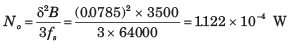QUESTION: 4

The SNR will be

Solution: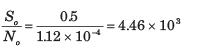QUESTION: 5

The output signal-to-quantization-noise ratio of a 10-bit PCM was found to be 30 dB. The desired SNR is 42 dB. It can be increased by increasing the number of quantization level.In this way the fractional increase in the transmission bandwidth would be (assume log210 = 0.3)

Solution: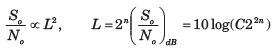= log C + 20 nlog2  =   α + 6 ndB. This equation shows that increasing n by one bits increase the by 6 dB.
Hence an increase in the SNR by 12 dB can be accomplished by increasing 9is form 10 to 12, the transmission bandwidth would be increased by 20%

QUESTION: 6

A signal has a bandwidth of 1 MHz. It is sampled at a rate 50% higher than the Nyquist rate and  quantized into 256 level using a  -low quantizer with μ = 225.

Que: The signal-to-quantization-noise ratio is

Solution: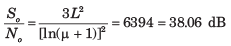QUESTION: 7

A signal has a bandwidth of 1 MHz. It is sampled at a rate 50% higher than the Nyquist rate and  quantized into 256 level using a  -low quantizer with μ = 225.

Que: It was found that a sampling rate 20% above therate would be adequate. So the maximum SNR, thatcan be realized without increasing the transmission bandwidth, would be

Solution:

Nyquist Rate = 2 MHz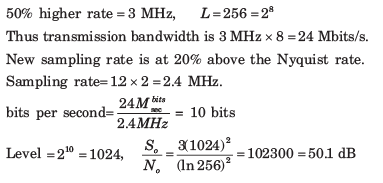QUESTION: 8

For a PCM signal the compression parameter μ = 100 and the minimum signal to quantization-noiseratio is 50 dB. The number of bits per sample would be.

Solution: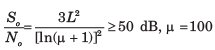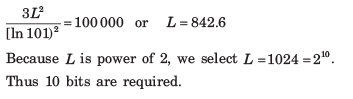QUESTION: 9

A sinusoid massage signal m(t) is transmitted by binary PCM without compression. If the signalto-quantization-noise ratio is required to be at least 48dB, the minimum number of bits per sample will be

Solution: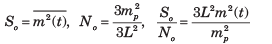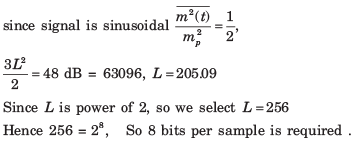QUESTION: 10

A speech signal has a total duration of 20 sec. It is sampled at the rate of 8 kHz and then PCM encoded. The signal-to-quantization noise ratio is required to be 40dB. The minimum storage capacity needed to accommodate this signal is

Solution: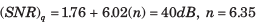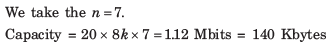QUESTION: 11

The input to a linear delta modulator having fstep-size Δ = 0.628 is a sine wave with frequency fm and peak amplitude Em. If the sampling frequency fs = 40 kHz, the combination of the sinc-wave frequency and the peak amplitude, where slope overload will take place is

Solution: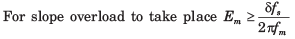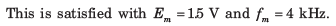QUESTION: 12

A sinusoidal signal with peak-to-peak amplitude of 1.536 V is quantized into 128 levels using a mid-rise uniform quantizer. The quantization-noise power is

Solution: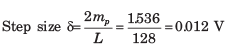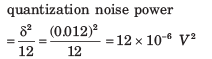QUESTION: 13

signal is sampled at 8 kHz and is quantized using 8 bit uniform quantizer. Assuming SNRq for a sinusoidal signal, the correct statement for PCM signal with a bit rate of R is

Solution:

Bit Rate = 8k x 8 = 64 kbps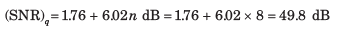QUESTION: 14

A 1.0 kHz signal is flat-top sampled at the rate of 180 samples sec and the samples are applied to an idealrectangular LPF with cat-off frequency of 1100 Hz, then the output of the filter contains

Solution: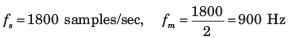Since the sampling rate is 1800 samples/sec the highest frequency that can be recovered is 900 Hz.

QUESTION: 15

The Nyquist sampling interval, for the signal sinc(700t) + sinc(500t) is

Solution:

x(t) = sinc 700t + sinc 500t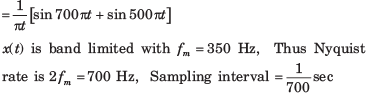QUESTION: 16

A signal x(t) = 100 cos(24π x 103)t is ideally sampled with a sampling period of 50 sec and then passed through an ideal lowpass filter with cutoff frequency of 15 KHz. Which of the following frequencies is/are present at the filter output

Solution: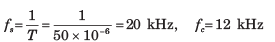The frequency passed through LPF are fc ,fs - fm or 12 kHz, 8 kHz

QUESTION: 17

In a PCM system, if the code word length is increased form 6 to 8 bits, the signal to quantization noise ratio improves by the factor.

Solution: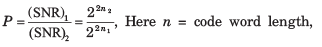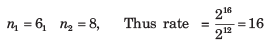QUESTION: 18

Four signals g1(t) , g3(t), g2(t) and g4(t) are to be multiplexed and transmitted. g1(t)  and g4(t) have a bandwidth of 4 kHz, and the remaining two signals have bandwidth of 8 kHz,. Each sample requires 8 bit for encoding. What is the minimum transmission bit rate of the system.

Solution:

signals g1(t) , g3(t), g2(t) and g4(t) will have 8 k, 8 k, 16 k and 16 k sample/sec at Nyquist rate. Thus 48000 sample/sec bit rate 48000 x  8 =384 kbps

QUESTION: 19

Three analog signals, having bandwidths 1200 Hz, 600 Hz and 600 Hz, are sampled at their respective Nyquist rates, encoded with 12 bit words, and time division multiplexed. The bit rate for the multiplexed signal is

Solution:

Analog signals, having bandwidth 1200 Hz, 600 Hz and 600 Hz have 2400, 1200 samples/sec at Nyquist rate. Hence 48000 sample/sec

bit rate  = 48000 sample/sec x 12 = 57.6 kbps

QUESTION: 20

The minimum sampling frequency (in samples/sec) required to reconstruct the following signal form its samples without distortion would be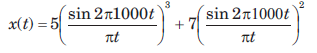Solution:Maximum frequency component = 3 x 1000 = 3 kHz Sampling rate = 2 fm = 6kHz